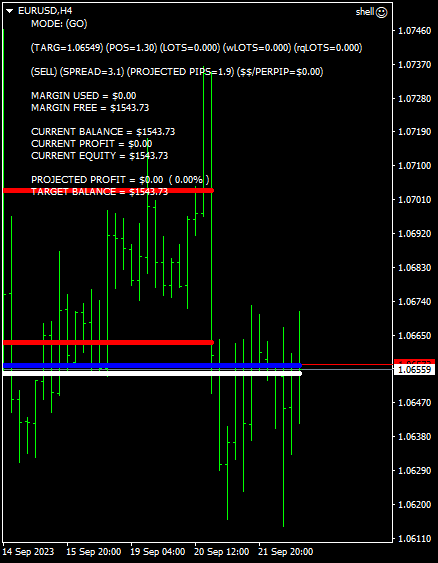I thought that i might try my hand at giving trading signals.

i also plan to post a note in “trader meetups”, in that i would like to establish a zoom chat with other like minded traders and risk analysts.

i tagged the senior moderator, so that i could avoid violating any of this website’s rules.

my trading bot does not use any “off the shelf” indicators or code, everything is custom.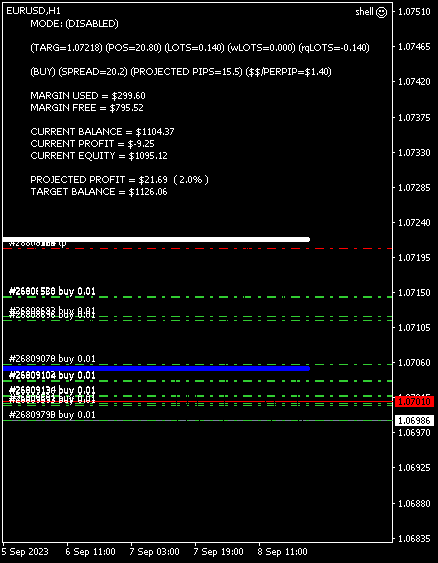this chart is current and displays my current LIVE TRADING.

BLUE LINE = BREAK EVEN
WHITE LINE = TARGET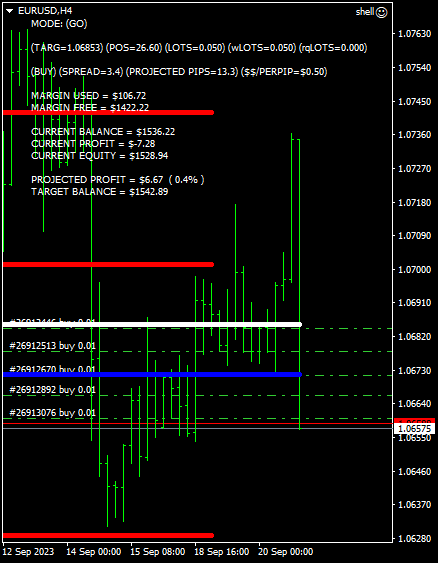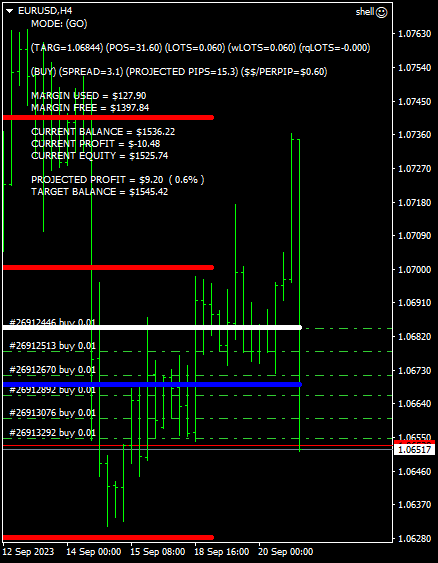BLUE LINE = BREAK EVEN
WHITE LINE = TARGET

RISK has been increased by a factor of three.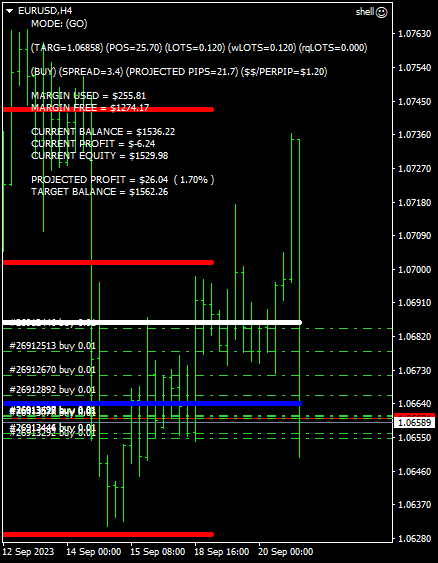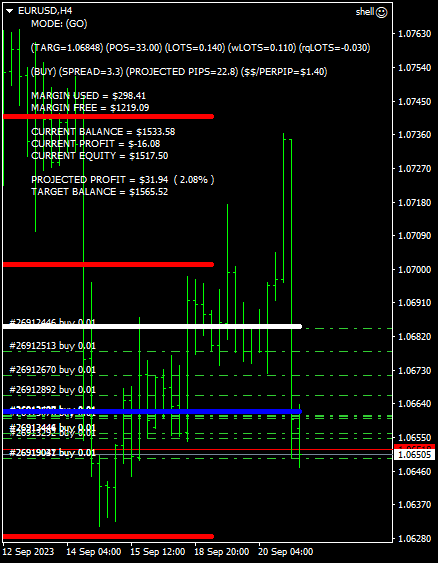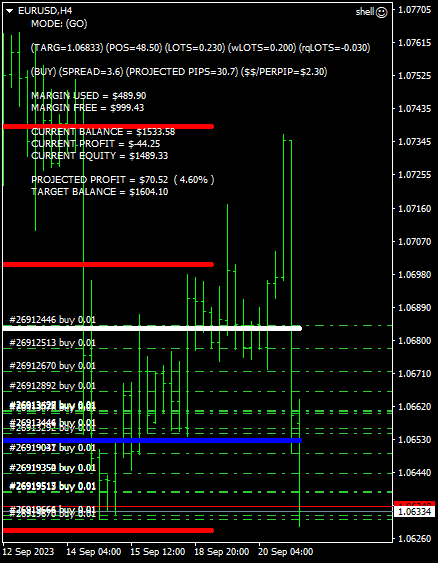previous position closed…

lets do it again.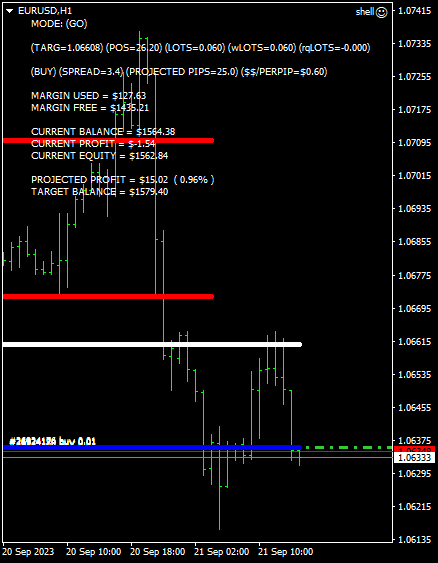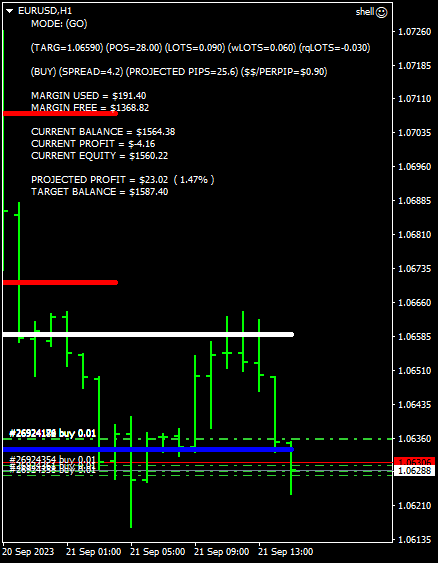i have two modes that my bot can operate in…

1. when i cross the target line(WHITE LINE) by one pip, it will block the trade by placing a takeprofit and stoploss on either side of the trade.

2. a takeprofit is automatically set at the target line(WHITE LINE) when any new trade is added to the position.

I am now trading in the second mode, takeprofit mode.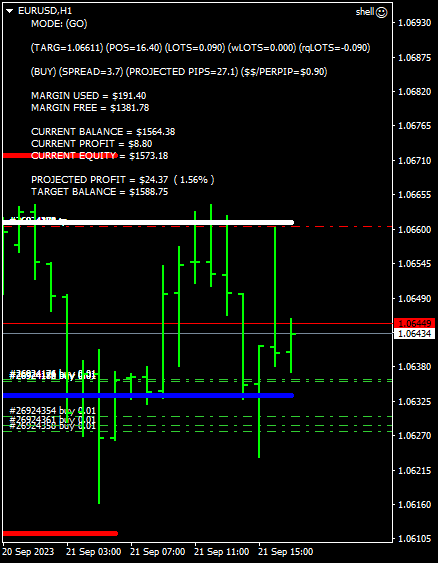position closed.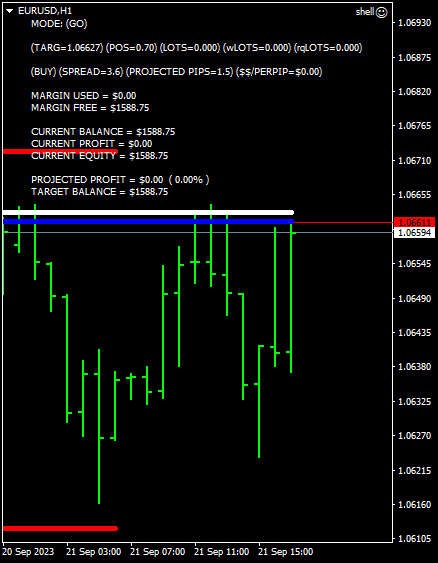new position planted.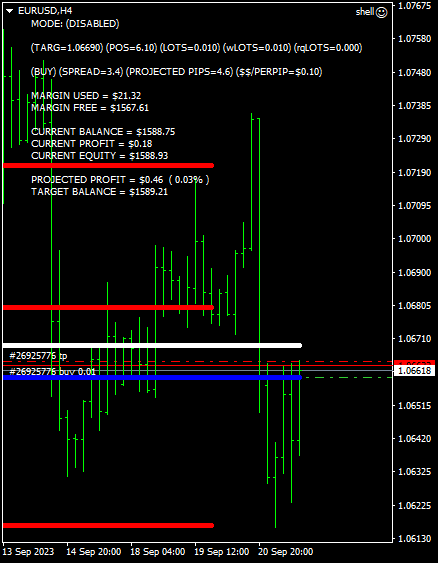``` double baselots=0.01; double mx=3; //gogo if(pos>=5) wantlots+=(baselots); if(pos>=10) wantlots+=(baselots); if(pos>=15) wantlots+=(baselots); if(pos>=20) wantlots+=(baselots*mx); if(pos>=25) wantlots+=(baselots*mx); if(pos>=30) wantlots+=(baselots*mx); if(pos>=35) wantlots+=(baselots*mx); if(pos>=40) wantlots+=(baselots*mx); if(pos>=45) wantlots+=(baselots*mx); if(pos>=50) wantlots+=(baselots*mx); if(pos>=55) wantlots+=(baselots*mx); if(pos>=60) wantlots+=(baselots*mx); if(pos>=65) wantlots+=(baselots*mx); if(pos>=70) wantlots+=(baselots*mx); if(pos>=75) wantlots+=(baselots*mx); if(pos>=80) wantlots+=(baselots*mx); if(pos>=85) wantlots+=(baselots*mx); if(pos>=90) wantlots+=(baselots*mx); ```
1. new position planted.

2. \$100.00 withdrawl requested.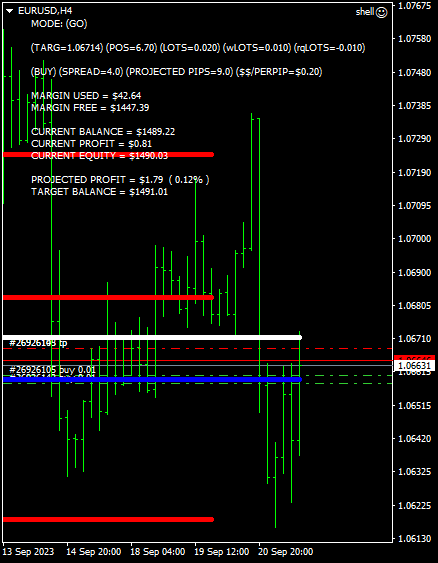position closed.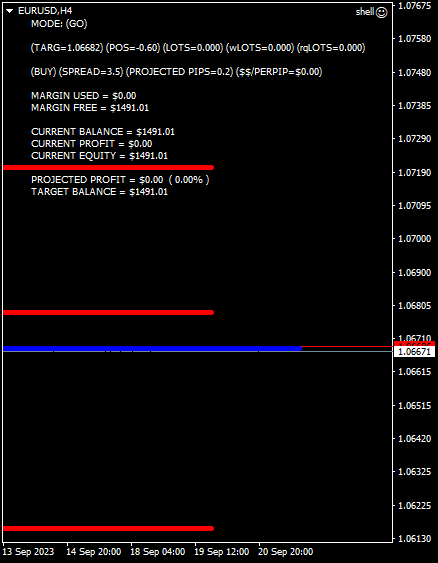new position planted.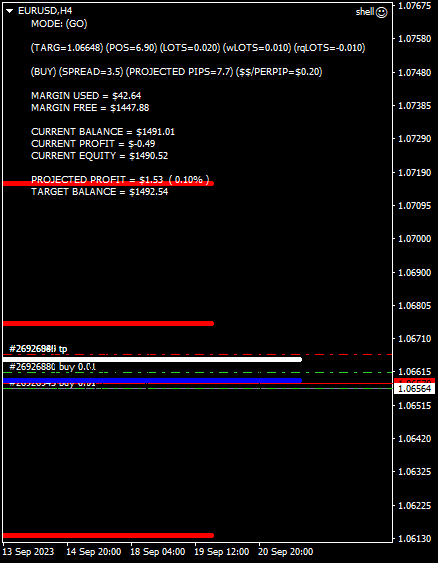risk algo changed.

``` double baselots=0.01; double wantlots=0; double mx=3; //gogo if(pos>=20) wantlots+=(baselots*mx); if(pos>=25) wantlots+=(baselots*mx); if(pos>=30) wantlots+=(baselots*mx); if(pos>=35) wantlots+=(baselots*mx); if(pos>=40) wantlots+=(baselots*mx); if(pos>=45) wantlots+=(baselots*mx); if(pos>=50) wantlots+=(baselots*mx); if(pos>=55) wantlots+=(baselots*mx); if(pos>=60) wantlots+=(baselots*mx); if(pos>=65) wantlots+=(baselots*mx); if(pos>=70) wantlots+=(baselots*mx); if(pos>=75) wantlots+=(baselots*mx); if(pos>=80) wantlots+=(baselots*mx); if(pos>=85) wantlots+=(baselots*mx); if(pos>=90) wantlots+=(baselots*mx); ```

position closed.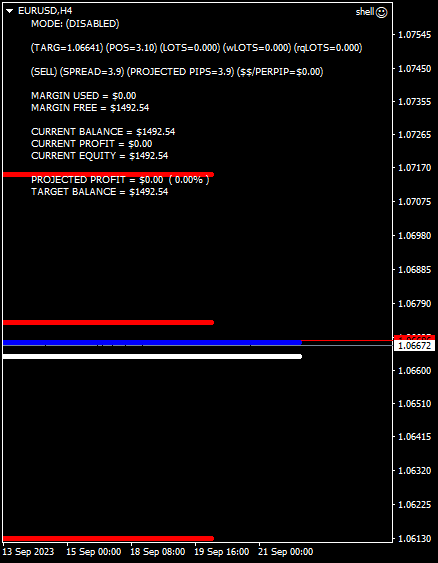giddyup, here we go again…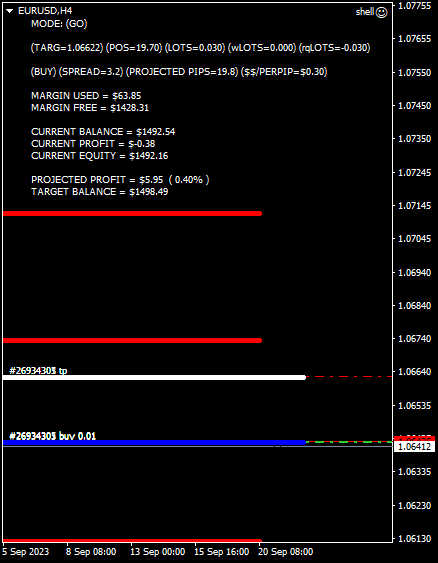position closed.oh my… i just walked out to check on my car and look what happened? somebody put all of these funny trades on my terminal.

i need to know if anybody knows the number for the forex police, because i am gonna press charges.

anyways, now position planted.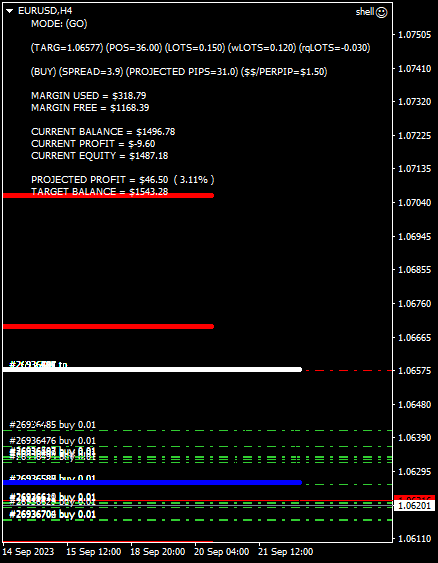this one close while i was hibernating(sleeping).